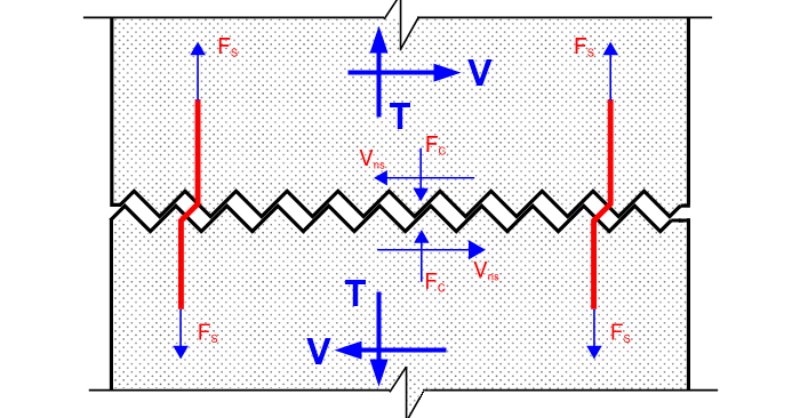# Understanding Out-of-Plane Shear Capacity of Concrete Wall Panels

When designing concrete shear walls for lateral loading, RISA-3D includes design checks for both in-plane and out-of-plane shear. In the condition where a concrete wall is under both axial tension and out-of-plane shear, the out-of-plane shear limit state for a specific region within the concrete wall detailed report includes the details shown below: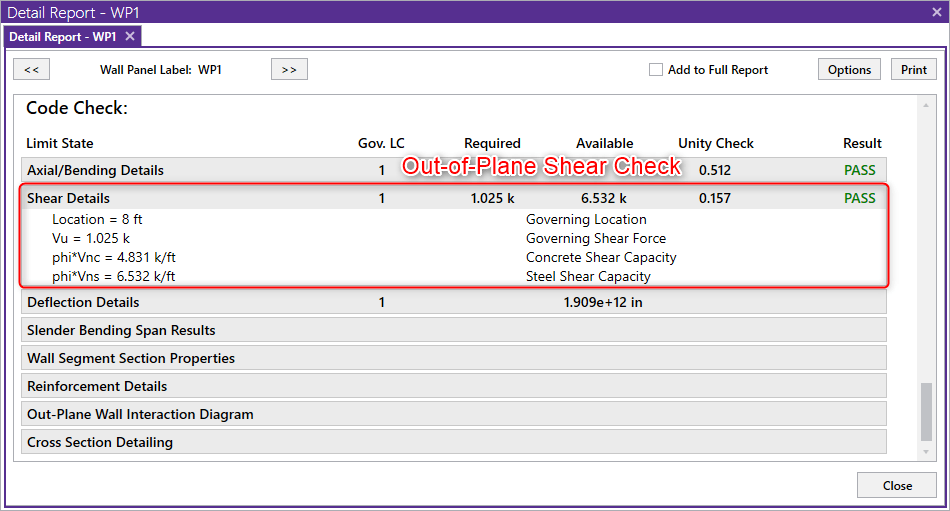This limit state may cause users to ask the question: “why is the a phi*Vns term listed when no out-of-plane shear reinforcement is present?”

The answer is that when calculating the out-of-plane shear capacity of the concrete wall, RISA-3D considers Shear Friction developed at the crack interface.

ACI 318 code defines shear friction as the shear transfer across any given plane, such as an existing or potential crack, an interface between dissimilar materials, or an interface between two concretes cast at different times. In this case, the existing/potential cracks condition applies.

When axial tension and out-of-plane shear are applied to the concrete wall, cracks will form across the wall thickness as explained by the simplified force diagram shown below: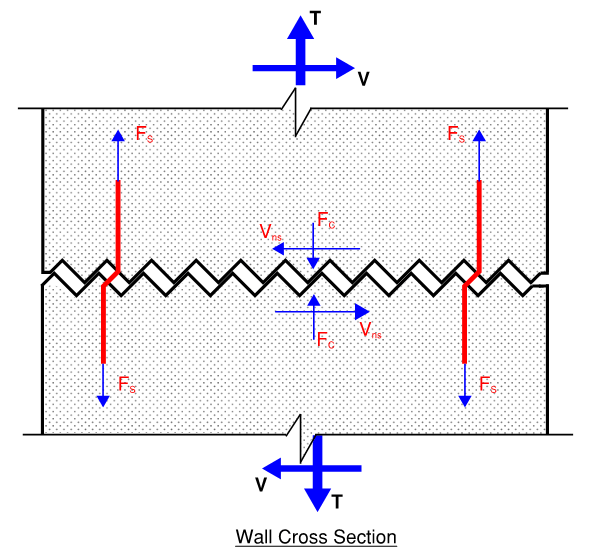The shear sliding at the crack generates tensile forces Fs in rebar. In return, these tensile forces, Fs cause compression forces, Fc at the concrete crack interface. The shear friction force (Vns) is described as the compression force multiplied by the coefficient of friction μ, where the compression force will be equal to the strength of the rebar at the ultimate stage.

According to ACI 318 Sec 22.9, RISA-3D defines shear strength due to shear friction as: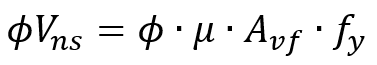where μ is assumed to be conservatively taken as 1.0 (see Table 22.9.4.2 in ACI 318-14). Moreover, the shear strength phi*Vns shall not exceed the shear strength without axial loads: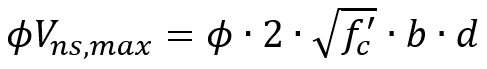Notice that some of the rebar, (Atension) is needed to resist the external tensile forces T. As a result, the rebar that can be used for shear friction (Avf) is a portion of the total rebar area As, given by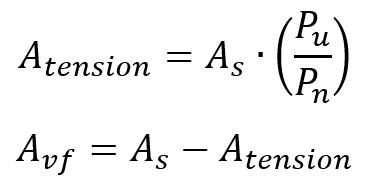where Pu is the external tensile force, and Pn is the tensile strength of the concrete wall.

In the code check section, the shear strength due to shear friction phi*Vns will be compared with the phi*Vnc calculated using ACI 318 Eqn. (22.5.7.1) with Nu being equal to the external tensile forces: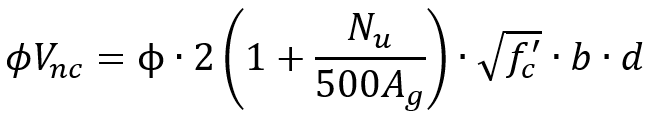The larger of phi*Vns and phi*Vnc will be used as the shear strength for the out-of-plane shear UC check. All the values used for the out-of-plane code check is on a per-foot basis.

On thing to note, if there is no axial tension in the wall then phi*Vns = 0 and shear friction will not be considered. The intention is to only use shear friction when needed for a case where the wall is loaded in tension and can produce a very small phi*Vnc value.